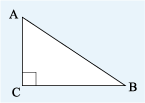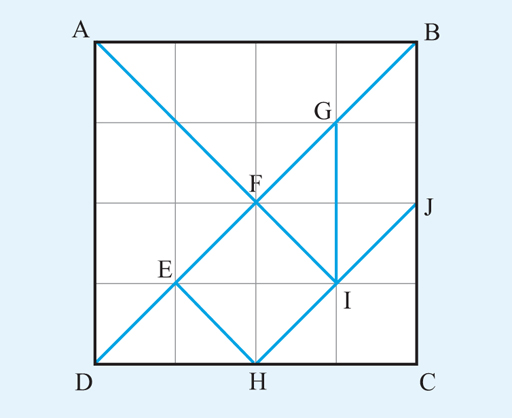Succeed with maths – Part 2

Start this free course now. Just create an account and sign in. Enrol and complete the course for a free statement of participation or digital badge if available.

Free course

# 1.4 Describing shapes

When you are describing a geometrical figure or shape, you often need to refer to a particular line or angle on the diagram, so others know what you are referring to. This can be done by labelling the diagram with letters. For example, Figure 10 shows a triangle labelled clockwise at the corners with A,B,C. This is then known as triangle ABC, in which the longest side is AB and the angle is a right angle. is the angle formed by the lines AC and CB. The point where two lines meet is known as a vertex (the plural is vertices). So A, B and C are vertices of the triangle.Figure 10 A triangle labelled ABC

Note that you can use the shorthand notation ‘’ for ‘the triangle ABC’ if you wish. There is a lot of new maths vocabulary in these last few sections, so you might find it useful to make a note of these to refer back to when completing this next activity, or for this week’s quiz and the badged quiz in Week 8.

## Activity 1 What can you see?

Timing: Allow approximately 10 minutes

Look at the image below and then answer the following questions using the letters shown.Figure 11
• a.Which sets of lines appear to be parellel?

• a.
• b.AB is parallel to DC.

AD is parallel to GI and BC.

AI is parallel to EH.

BD is parallel to HJ

• b.Which lines are perpendicular?

• b.AB is perpendicular to AD and to BC.

DC is perpendicular to AD and BC.

EH is perpendicular to DB and HJ.

AI is perpendicular to HJ and DB.

• c.How many triangles can you see?

• c.
• d.What other shapes can you see?

• d.The parallelogram, GBJI.

The squares, ABCD and EFIH.

The trapeziums, DHIF, EHIG, DBJH, BJHE and DGIH.

This completes your work on defining shapes and how to label them in order to describe them clearly to others.

The next section looks at the different ways for measuring shapes.